## Discovering Group Theory, Day 20

On Day 20, after reviewing the Galilean group and the classic formula for the addition of velocities, we went on to talk about their incompatibility with the results of the Michelson–Morley experiment. We begun to resolve the issue by deriving the expression for time dilation using the example of a moving light clock.Light clock

## Color Physics @Hampshire, Day 21

On Day 21, we first reviewed the interaction of light and matter. Students then used hand-held spectrometers to observe and record the spectrum of hydrogen and identified the mystery gas in one of the tubes.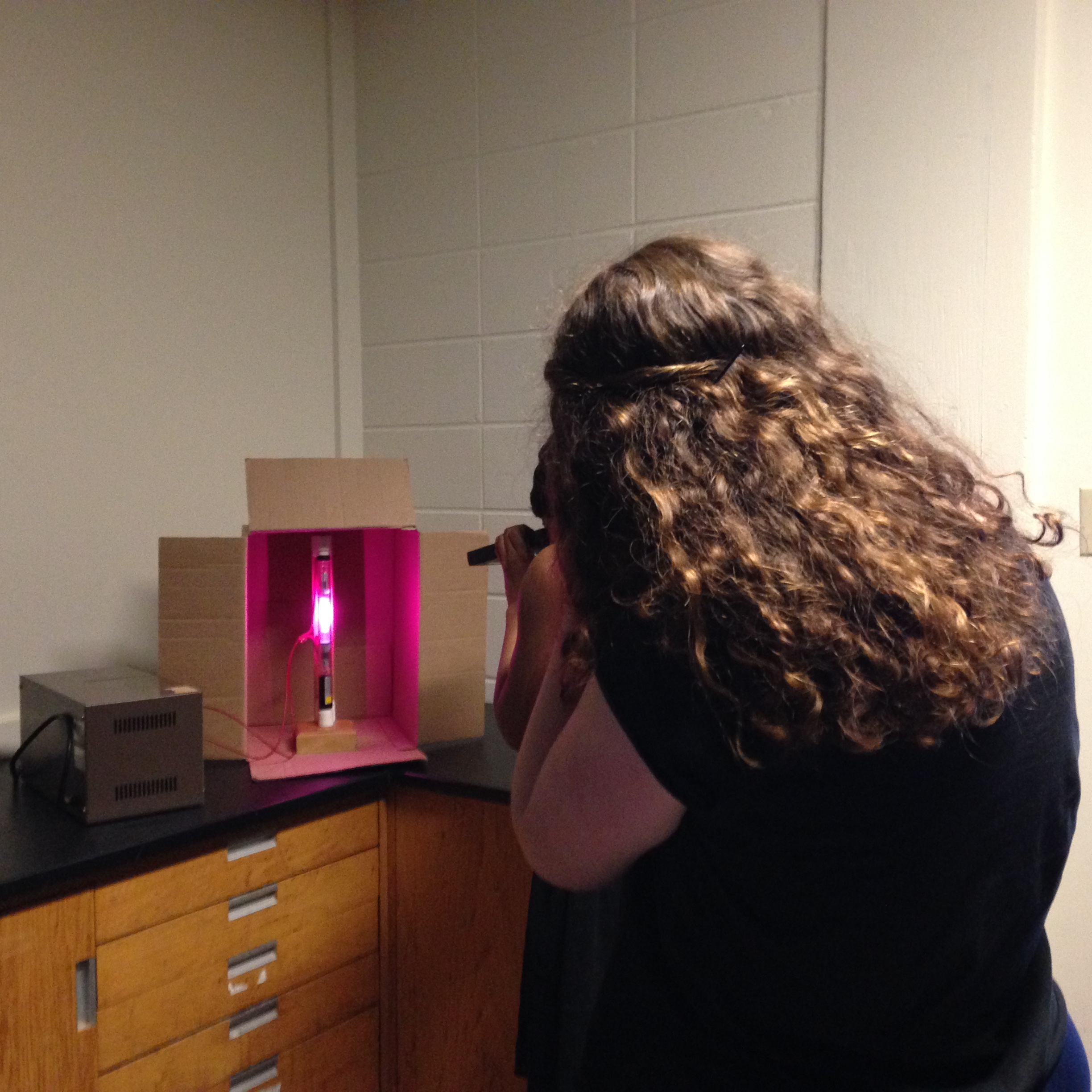Students used hand-held spectrometers to observe and record spectra of two gases.

## Color Physics @Hampshire, Day 20

On Day 20, we covered two different topics.

We first discussed how reflection and refraction affect the saturation of pigments.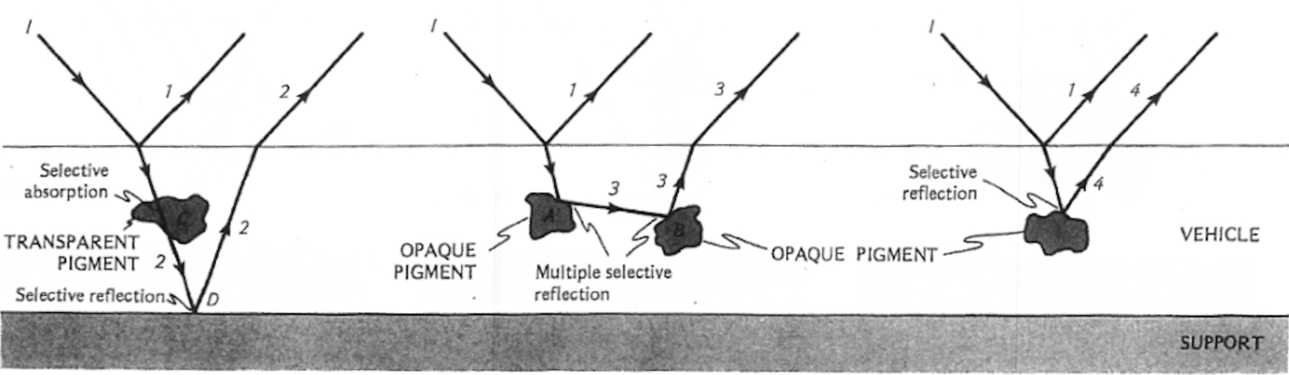Reflection and Refraction in pigments from Seeing the Light: Optics in Nature, Photography, Color, Vision, and Holography by David R. Falk, Dieter R. Brill , David G. Stork. Wiley, 1986.

We then moved on to some basic quantum mechanics in order to explain why various pigments absorb and reflect different wavelengths. We used a simple model of atom energy levels of sodium. New concepts introduced included

• Photons and their energy E=hc/λ
• Quantized atomic energy levels
• Energy levels of sodium
• Emission and absorption spectra of gases

## Color Physics @Hampshire, Day 19

On Day 19 we studied reflection and refraction. Students used light sticks, mirrors, and acrylic blocks to investigate, both qualitatively and quantitatively, the optical behavior of light at the interface of material with different indices of refraction. Their investigations led us to the law of reflection and Snell’s law.*

We introduced a number of new concepts:

• index of refraction
• normal to the surface
• angle of incidence
• angle of reflection
• angle of refraction/transmitted angle

*The photgraphs taken by and used with the permission of Andrew Hart of Hampshire College.

## Color Physics @Hampshire, Day 18

On Day 18 we

• Discussed the pieces students drew/painted over the course of the last three daysA halftone exercise with watercolors by Madeleine Perreault.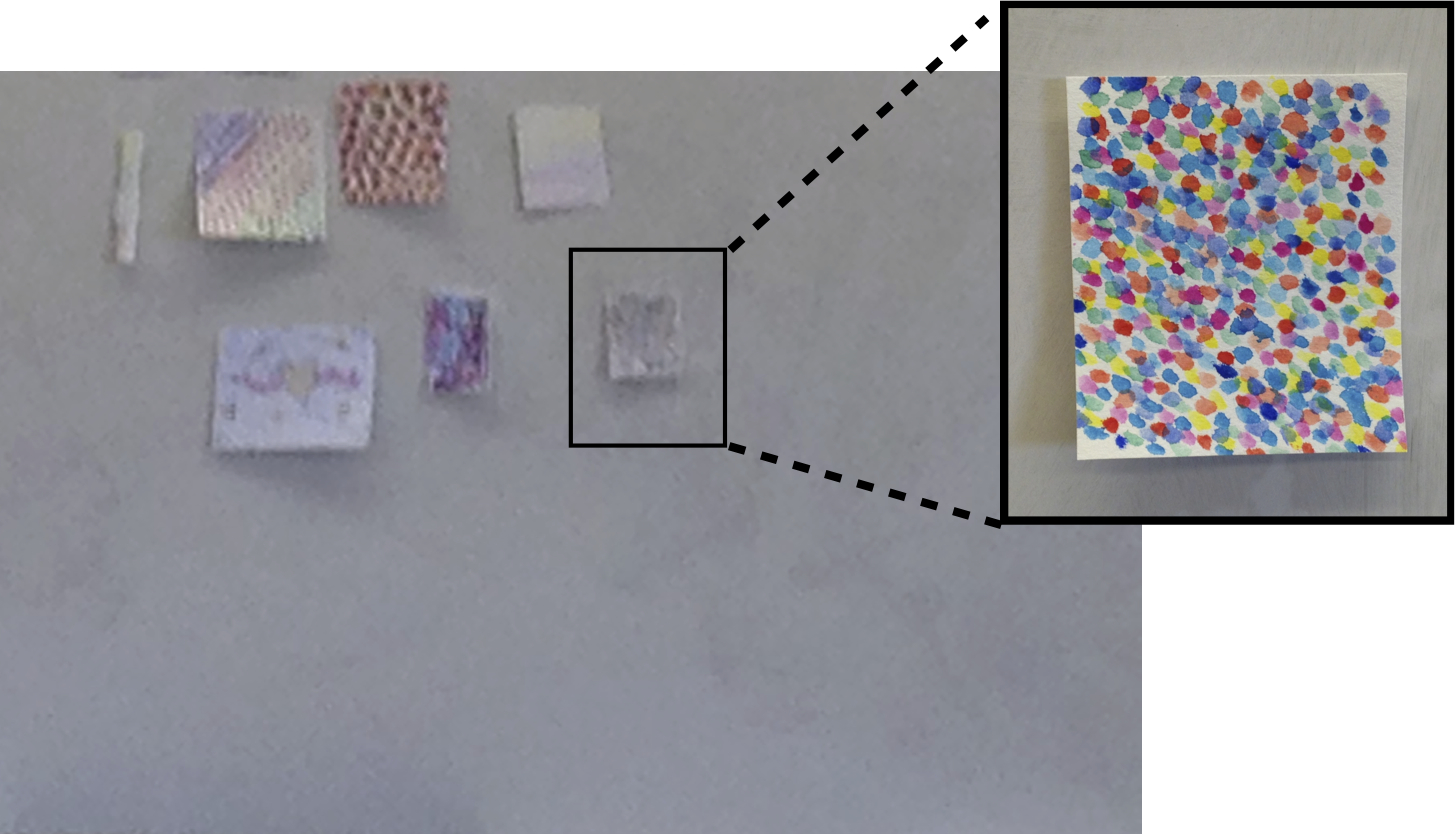The effects of additive mixing. Piece by Sara Young, looks grey from far away, but up close it is a colorful mixture.
• Practiced how to determine the spectral composition of reflected light from the spectral composition of incident light and the reflectance curves of the surfaces.Given date for a sample problem for which students used the incident intensity distribution and the reflectance curve to find the intensity distribution of the reflected light.

## Discovering Group Theory, Day 19

For this portion of the course, my key goal is that students understand  how group theory is used in physics, so that they can pursue the subject further if it catches their interest.

In preparation for day 19, students were asked to work through Khan Academy videos on 2×2 matrices outside of class

For most students, this was a review, but some of them have not seen matrices before. Rather than introducing everything about matrices, we only discussed those operations needed to prove that rotation matrices form a group SO(2) under matrix multiplication.

While proving that the set of 2×2 rotation matrices is closed under matrix multiplication, we used our recently acquired knowledge of complex numbers to derive the equations for cosine and sine of the sum of the angles.We introduced SO(3) by analogy to SO(2), but without going into the technical details.

After wrapping up rotations, we then moved on to moving frames of reference and the Galilean principle of relativity. We showed that acceleration is the same in all frames of reference moving at constant velocity relative to one another. This calculation required the understanding of a derivative, which was familiar to most students but not all, so I emphasized the conceptual understanding. We then defined Galilean group.Galilean relativity

To create excitement for what is to come, I briefly reiterated the physical interpretation of the law of addition of velocities, which we derived in the above-discussed calculation, and introduced the contradiction between it and the observed constant speed of light in vacuum.

## Discovering Group Theory, Day 18

On Day 18, we returned to the spacetime symmetries we discussed last time, but treated them with a bit more rigor and tighter notation.  In the context of the Newton’s 2nd law, we worked out the math for

• Translation group as applied to three-dimensional space and to time.
• Rotations in space and their matrix representation in 2D.
• We formally defined the orthogonal group O(2) and the special orthogonal group SO(2).

## Discovering Group Theory, Day 17

Realizing that space-time symmetries provide more intuitive introduction to the abstract notion of a symmetry used in physics,  on day 17 we switched gears by discussing Richard Feynman’s lecture “Symmetry in Physical Law,” which students watched outside the class.

Feynman introduces the notion of a symmetry of a physical law, which we disected in the context of Coulomb’s law.

• We defined a coordinate system and discussed the difference between passive and active transformations.
• We worked out the translational and rotational spatial symmetries in 2D and showed that translations form a group.
• We also introduced translations in time and showed how they leave the kinematic equations unchanged.

As a transition to the discussion of moving observers, we watched the first half of a wonderful video from the 60’s titled Frames of Reference.

## Discovering Group Theory, Day 16

On Day 16, we finished up complex numbers in polar form and introduced several new concepts:

• The circle group T and unitary group U(1)
• Infinite vs finite groups
• Continuous vs discrete groups

We also started going through t’Hooft’s Scientific American article “Gauge Theories of the Forces between Elementary Particles“, which proved to be challenging but exciting. We discussed the Standard Model of particle physics and the distinction between spacetime symmetries and internal symmetries.

## Color Physics @Hampshire, Days 15-17

Days 15, 16, and 17 were spent on hands-on activities in which students investigated subtractive mixing by reflection, and explored the use of additive and subtractive mixing in print and painting. The activities included:

• Creating partitive mixtures in the pointillist style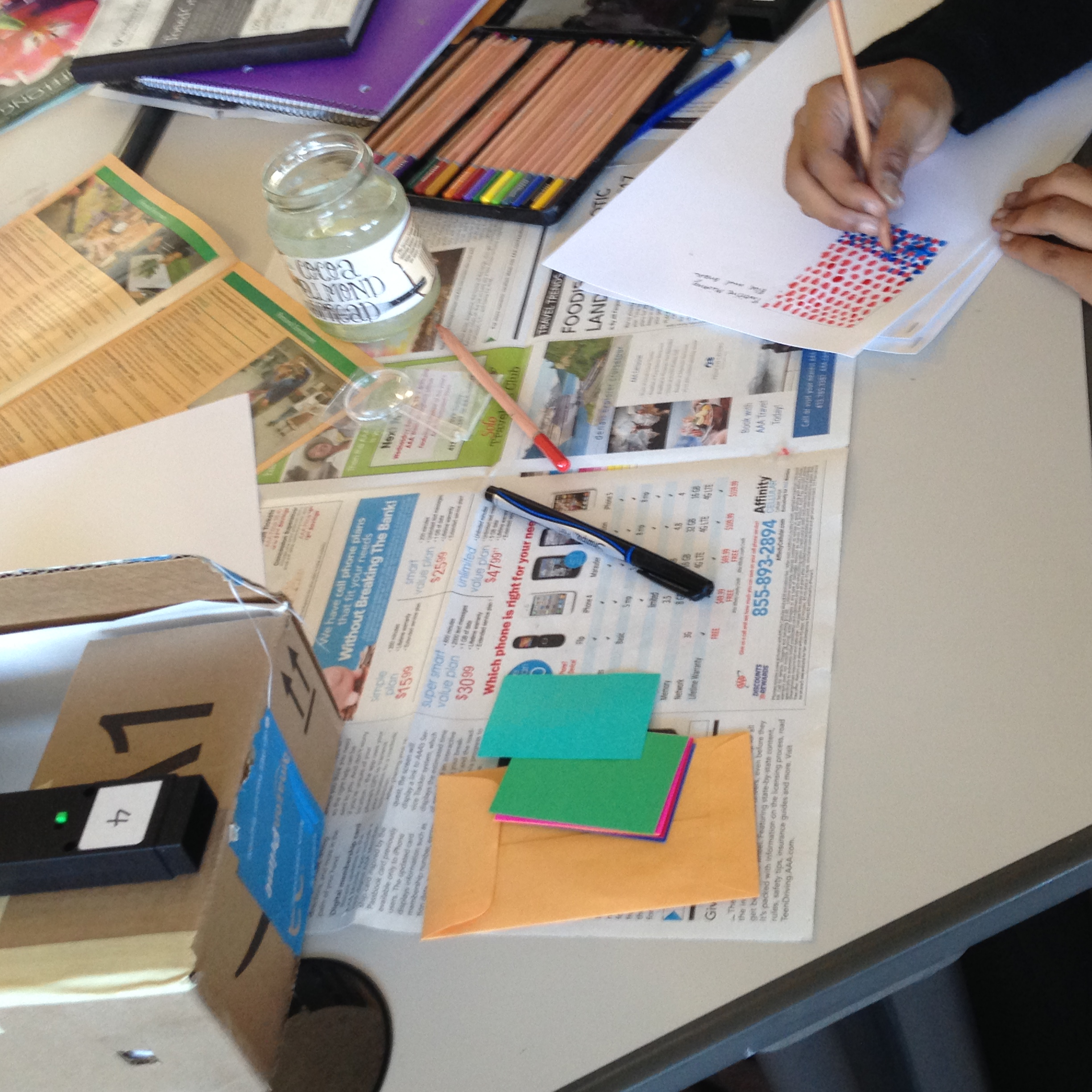• Investigating and replicating the use of halftone in print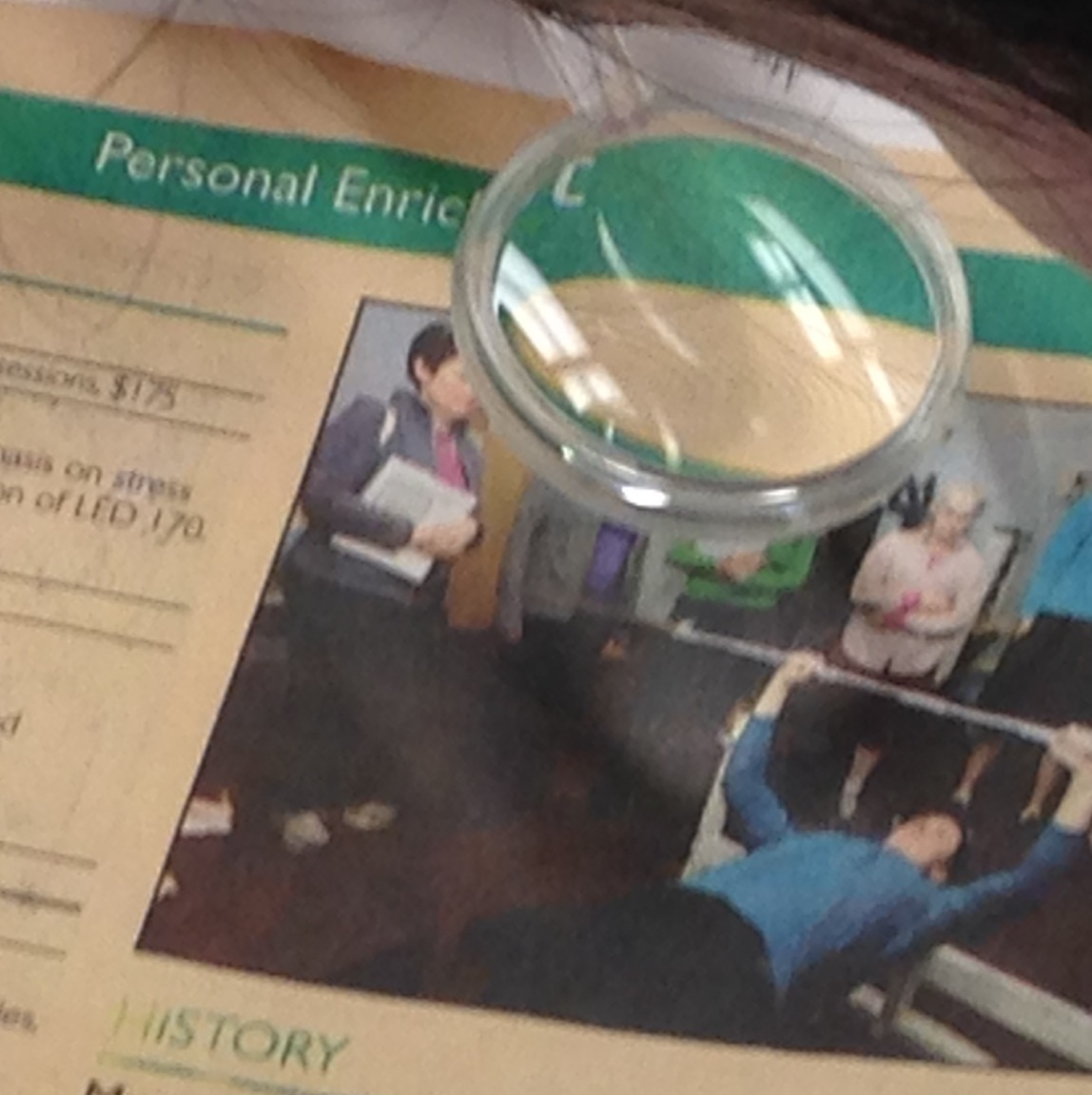Students used magnifying glasses to look at newsprint and determined how the CMYK system is used for printing. Then the made their own halftone designs.

• Investigating subtractive mixing by reflection.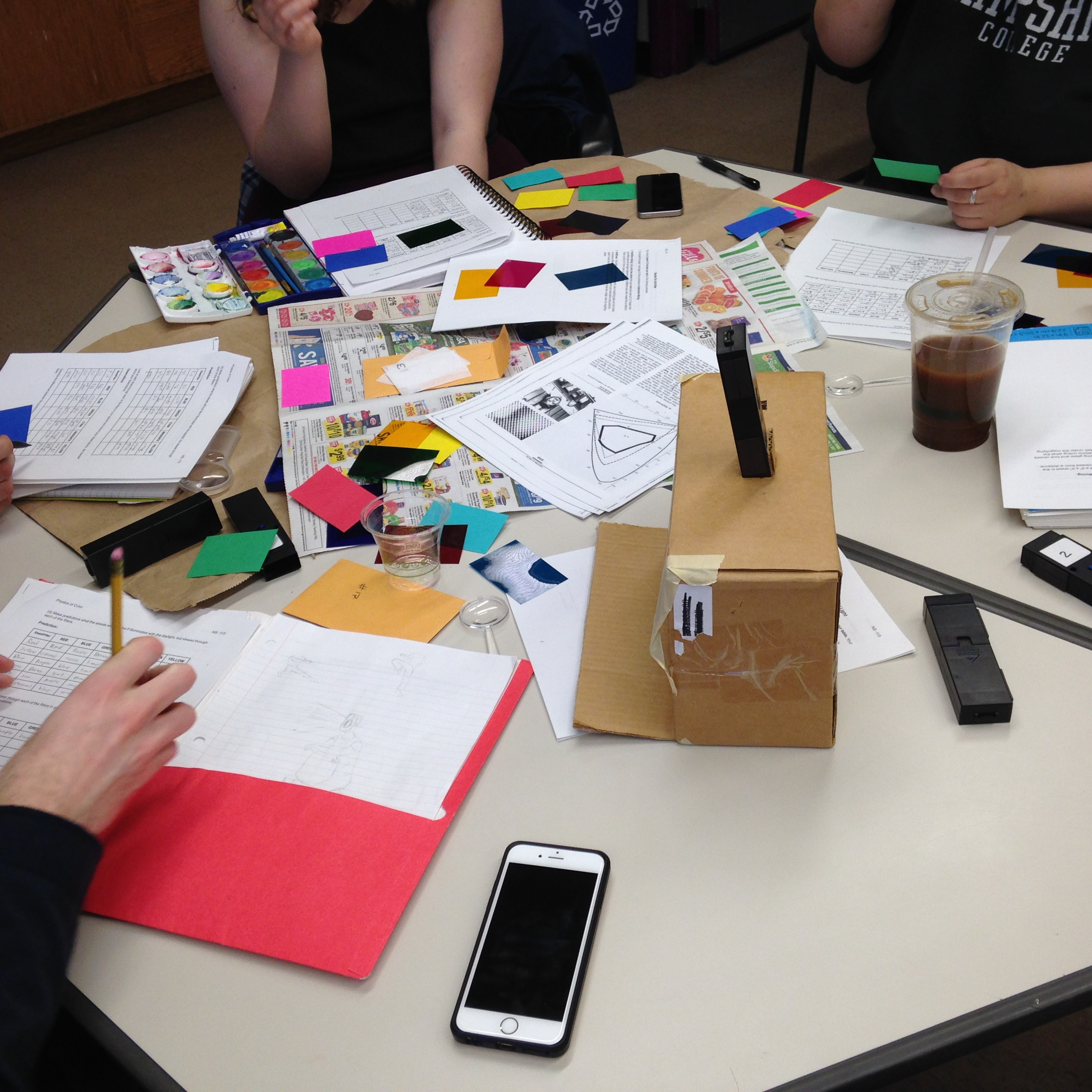This portion involved three different activities:
• In the first, students placed pieces of paper of various colors in a light-tight box,  illuminated them by one of the lights we have (red, green, or blue), and recorded their observations. The papers we used were to an OK approximation, red, blue, green, cyan, magenta, and yellow.
• In the second activity, students used their observations from the first to predict what colors the same pieces of paper would appear to have when illuminated by white light, but viewed through filters of different colors. They then tested their predictions and commented on any discrepancies.
• Finally, using what they learned from the first two activities, students devised a method by which they can determine how “pure” the cyan, magenta, and yellow pigments in their watercolor sets were.A student-made hologram of a thread spool